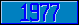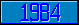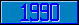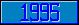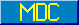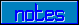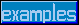## \$%LOG10^MATH

### M[UMPS] by Example

Approved for inclusion in a future M[UMPS] language standard as part of the library for General Mathematics.

This function returns the 10-based logarithm of the value of its parameter.

Reference Value \$%LOG10^MATH(1) 0 \$%LOG10^MATH(10) 1 \$%LOG10^MATH(100) 2 \$%LOG10^MATH(\$%E^MATH(),7) .4342945

The MDC has approved code to approximate the return value of this function. Implementors are encouraged to provide more accurate and efficient code).